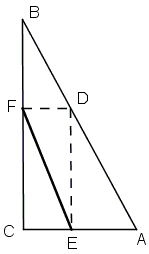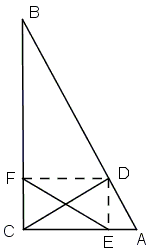Distance Between Projections

Here are two apparently similar problems. In the very least, both have solutions that stem from the same basic reasoning.

Problem 1

E and F are projections of point D on the hypotenuse of right triangle ABC on legs AC and BC, respectively. Find D for which EF is minimal.Problem 2

E and F are projections of point D on a quarter circle arc AB on the axes, respectively. Find D for which EF is minimal.SolutionSolutions

Problem 1

E and F are projections of point D on the hypotenuse of right triangle ABC on legs AC and BC, respectively. Find D for which EF is minimal.

Draw the second diagonal in rectangle CEDF. Two diagonals in a rectangle are equal. It follows that EF will be shortest whenever CD is. But CD joins vertex C of ΔABC to the opposite side AB. CD is the shortest when it's the altitude from C.Problem 2

E and F are projections of point D on a quarter circle arc AB on the axes, respectively. Find D for which EF is minimal.

By the same reasoning as in Problem 1, EF is the shortest when the other diagonal CD is. But CD is the radius of the quarter circle and does not depend on theposition of D. The same holds for EF: EF is constant independent of the position of D on the circle.(There is a classical problem by Franciscus van Schooten, related to Problem 2.)A Sample of Optimization Problems III

• Mathematicians Like to Optimize
• Mathematics in Pizzeria
• The Distance to Look Your Best
• Building a Bridge
• Linear Programming
• Residence at an Optimal Distance
• Huygens' Problem
• Optimization in a Crooked Trapezoid
• Greatest Difference in Arithmetic Progression
• Area Optimization in Trapezoid
• Minimum under Two Constraints
• Optimization with Many Variables
• Minimum of a Cyclic Sum with Logarithms
• A Problem with a Magical Solution from Secrets in Inequalities
• Leo Giugiuc's Optimization with Constraint
• Problem 4033 from Crux Mathematicorum
• An Unusual Problem by Leo Giugiuc
• A Cyclic Inequality With Constraint in Two Triples of Variables
• Two Problems by Kunihiko Chikaya
• An Inequality and Its Modifications
• A 2-Variable Optimization From a China Competition
•